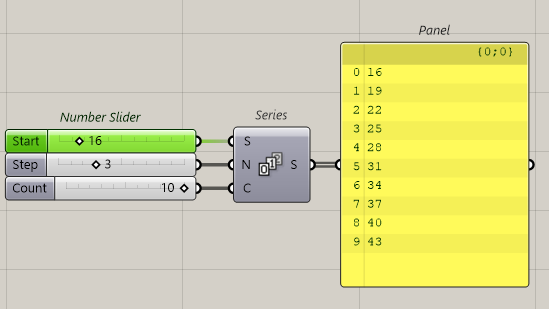# Expression Calculating

Hello.
Need to fillet some edges (number of edges changes dynamically). I have to provide edge number(s) as a list. How to calculate this list, if edge number changes like 16 + x*3, where x changes from 0 to number of edges. So if number of edges will change (less or more), the list shoud change too. How to calculate those values and provide them as a list or like so?

Hi , use series:1 Like

Exactly what I need! Thank you so much!
I was searching for a loop and couldn’t find it.